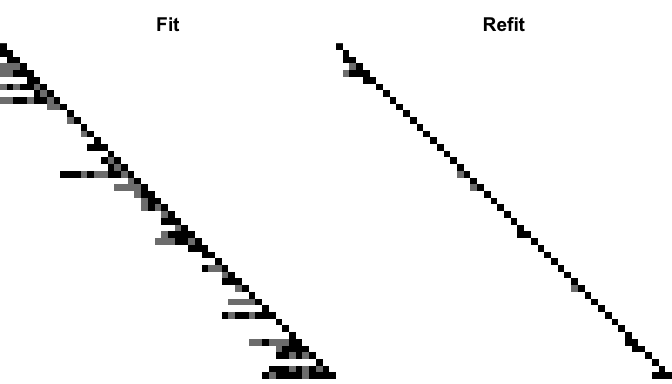Using the varband package

2016-11-07

The varband package contains the implementations of the variable banding method for learning local dependence and estimating large sparse precision matrix in the setting where variables have a natural ordering. The details of the method can be found in Yu, Bien (2016) Learning Local Dependence in Ordered Data(under revision). In particular, given a data matrix $$X \in \mathbb{R}^{n \times p}$$, with each row an observation of a $$p$$ dimensional random vector $$X \sim N(0, \Omega^{-1} = (L^T L)^{-1})$$, this package implements a penalized likelihood-based approach of estimating $$L$$ with data-adaptively variable bandwidth. This document serves as an introduction of using the package.

The main function is varband, which takes a sample covariance matrix of the observations and returns the estimate of $$L$$. For demonstration purpose and simulation study, the package also contains functions to generate random samples from true models with user-specified variable banded patterns.

Data simulation

The package contains two functions for generating true models: ar_gen and varband_gen. The function ar_gen takes a vector of pre-specified off-diagonal values and returns a strictly banded $$L$$, which corresponds to a autoregressive model of order equal to the bandwidth. The function varband_gen returns a lower triangular block-diagonal matrix with each block having variable bandwidth.

library(varband)
set.seed(123)
p <- 50
n <- 100
true <- varband_gen(p = p, block = 5)

With a generated true model in place, we can then generate a data matrix $$X \in \mathbb{R}^{n \times p}$$ with each row a random sample drawn independently from a Gaussian distribution of mean zero and covariance $$\Sigma = (L^T L)^{-1}$$.

# random sample
x <- sample_gen(L = true, n = n)
# sample covariance matrix
S <- crossprod(scale(x, center = TRUE, scale = FALSE)) / n

And we can plot the sparsity patterns of the true model and the sample covariance matrix by using matimage

par(mfrow = c(1, 2), mar = c(0, 0, 2, 0))
matimage(true, main = "True L")
matimage(S, main = "Sample covariance matrix")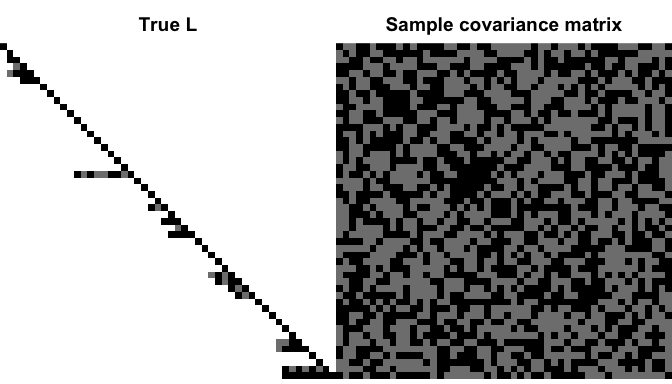Estimating $$L$$ with a fixed tuning parameter

Besides the sample covariance matrix, the main function varband takes three more arguments. First it takes a value of the tuning parameter $$\lambda$$, which is a nonnegative constant that controls the sparsity level induced in the resulting estimator. The function also requires an initial estimate, which could essentially be any lower triangular matrix with positive diagonals. Finally one needs to specify the weighting scheme w to choose between a weighted and an unweighted estimator. The unweighted estimator puts more penalty and thus produces a sparser estimator than the weighted one with the same value of $$\lambda$$. As shown in the paper, the unweighted estimator is more efficient to compute and has better practical performance, while the weighted estimator enjoys better theoretical properties.

# use identity matrix as initial estimate
init <- diag(p)
L_weighted <- varband(S = S, lambda = 0.4, init = init, w = TRUE)
L_unweighted <- varband(S = S, lambda = 0.4, init = init, w = FALSE)
par(mfrow = c(1,2), mar = c(0, 0, 2, 0))
matimage(L_weighted, main = "weighted, lam = 0.4")
matimage(L_unweighted, main = "unweighted, lam = 0.4")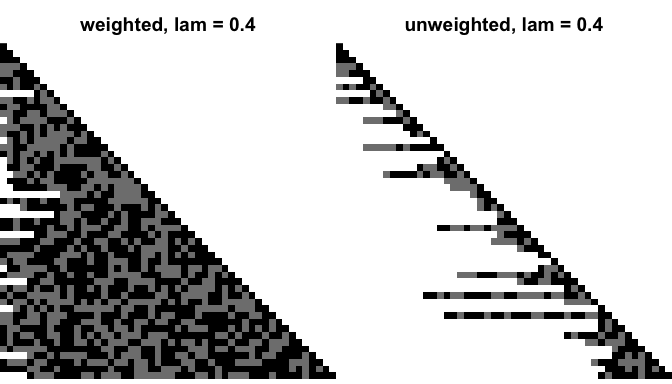Computing estimators along the a tuning parameter path

In most cases, one does not know the exact value of tuning parameter $$\lambda$$ that should be used. The function varband_path gets $$\hat{L}$$ along a grid of $$\lambda$$ values. Users can specify their own grid of $$\lambda$$ values (via lamlist). Alternatively, a path of decreasing tuning parameter of user-specified length (via nlam) will be generated and returned. In this situation, user also needs to specify flmin, the ratio of the smallest and largest $$\lambda$$ value in the list, where the largest $$\lambda$$ is computed such that the resulting estimator is a diagonal matrix. And we can plot them to see if they cover the full spectrum of sparsity level.

# generate a grid of 40 tuning paramters,
# with the ratio of smallest value and largest value equals to 0.03
res <- varband_path(S = S, w = FALSE, nlam = 40, flmin = 0.03)
par(mfrow = c(8, 5), mar = 0.1 + c(0, 0, 2, 0))
for (i in seq_along(res$lamlist)) matimage(res$path[, , i], main = sprintf("lam=%s", round(res$lamlist[i], 4)))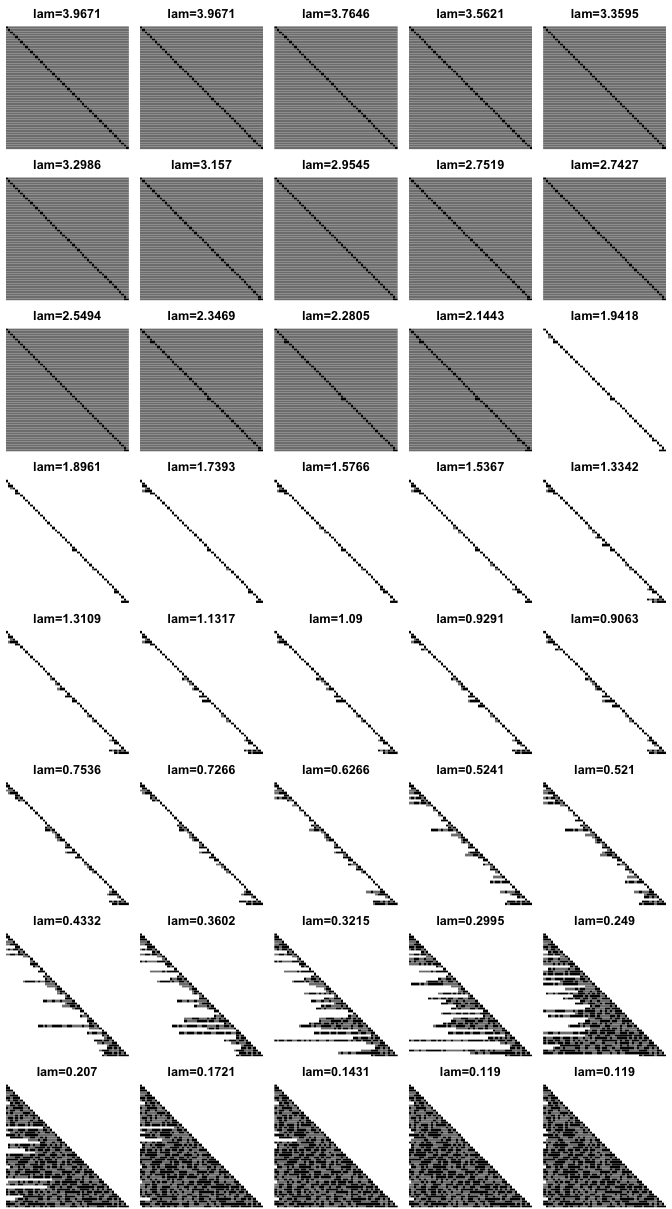Selecting the tuning parameter User can also use an implementation of cross-validation process(in varband_cv) to select the best value for tuning parameter. The cross-validation selects the value for lambda such that the resulting estimators attains the highest average likelihood on the testing data. res_cv <- varband_cv(x = x, w = FALSE, nlam = 40, flmin = 0.03) m <- rowMeans(res_cv$errs_fit)
se <- apply(res_cv$errs_fit, 1, sd) / sqrt(length(res_cv$folds))
plot(res_cv$lamlist, m, main = "negative Gaussian log-likelihood", xlab = "tuning parameter", ylab = "average neg-log-likelihood", type="o", ylim = range(m - se, m + se), pch = 20) # 1-se rule lines(res_cv$lamlist, m + se)
lines(res_cv$lamlist, m - se) abline(v = res_cv$lamlist[res_cv$ibest_fit], lty = 2) abline(v = res_cv$lamlist[res_cv$i1se_fit], lty = 2)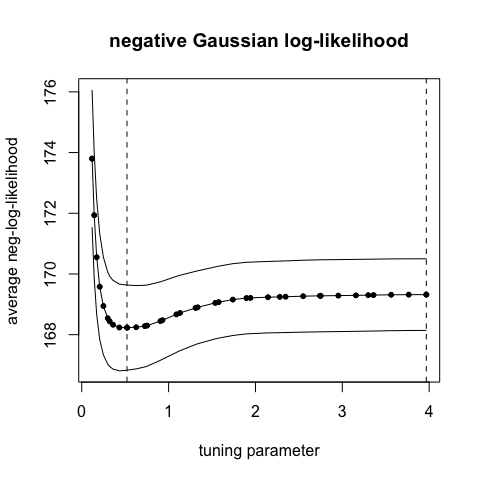The return of varband_cv is a list of many objects. For details see ?varband_cv. In particular, the function also returns the best refitted version of estimates. For example, to take a look at the support of the best refitted estimate, use par(mfrow = c(1,2), mar = c(0, 0, 2, 0)) matimage(res_cv$L_fit, main = "Fit")
matimage(res_cv\$L_refit, main = "Refit")